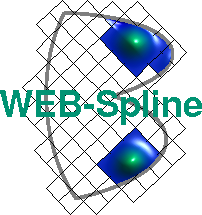# Membrane Problem

The domain in the following Figure is modeled by four non-uniform rational B-spline curves (NURBS).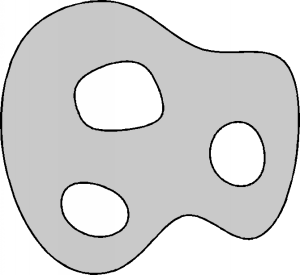It represents an irregularly shaped membrane, which is fixed at the boundary and subjected to constant vertical pressure.

The displacement u(x,y) is the solution of the following boundary value problem: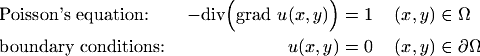The boundary conditions are essential and modeled with a weight function. Because of the irregularly shaped boundary it is constructed numerically with the aid of the distance function depicted below.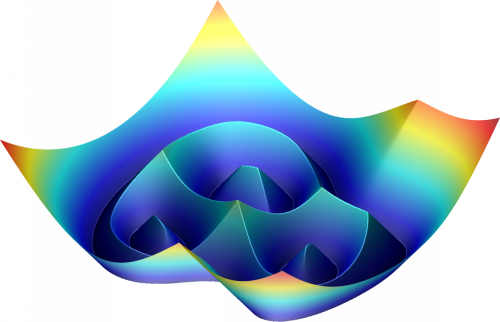To obtain a smooth distance function we blend the Euclidian distance with a plateau of height one in a strip near the boundary. The resulting weight function is shown below.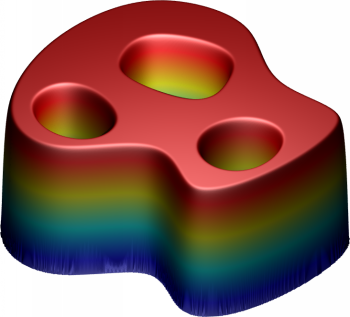The next Figure shows the displacement (considerably magnified), which was computed with cubic WEB-splines.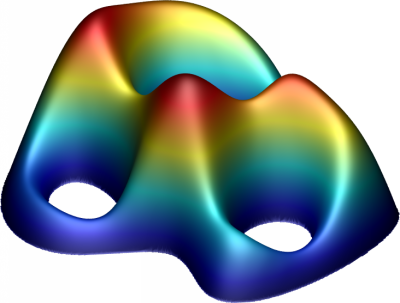### Literature

K. Höllig, U. Reif, J. Wipper: Weighted extended b-spline approximation of Dirichlet problems. SIAM Journal on Numerical Analysis, Volume 39, Number 2, pp. 442-462, 2001.

See Publications for a complete list of WEB-publications.

Author: Joachim Wipper ; Last modification: 2006/08/31 09:37:55 UTC.

 [Home] [The WEB-Method] [Examples] [Publications] [Contact]